# Please show all work: Let P1 = 1 If x is odd then Px+1 = 2Px...

Let P1 = 1

If x is odd then Px+1 = 2Px

If x is even then Px+1 = 2Px +1

Prove that 2Px+1 + 2Px+1 +1 = Px+2 is true and then solve it.

First note that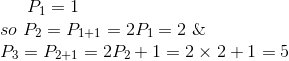But if the given relation were true then for x=1, we should have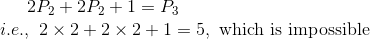.

Rather by definition of P, it will satisfy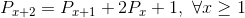. Let us prove this.

If x=2k, even, then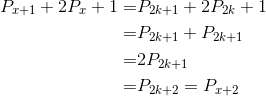.

If x=2k-1, odd, then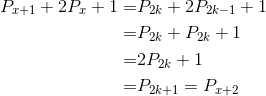.

Now to solve the relation.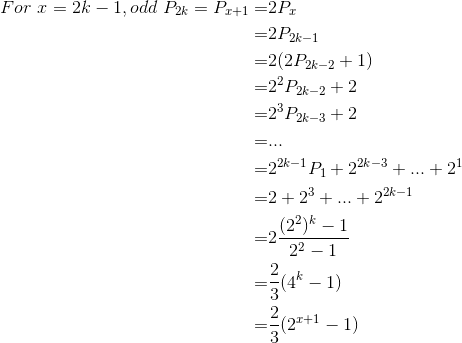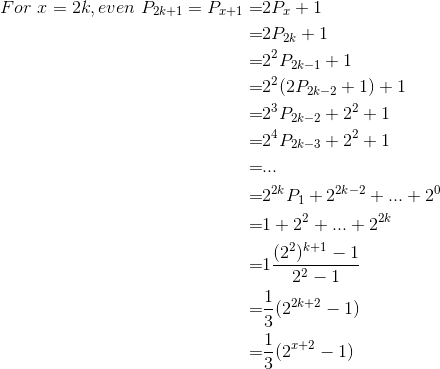Hence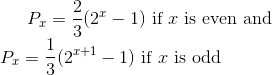##### Add Answer of: Please show all work: Let P1 = 1 If x is odd then Px+1 = 2Px...
Similar Homework Help Questions
• ### Let P1 = 1 If x is odd then Px+1 = 2Px If x is even...

Let P1 = 1 If x is odd then Px+1 = 2Px If x is even then Px+1 = 2Px +1    Show this is true and solve it: 2Px+1 + 2Px+1 +1 = Px+2

• ### Please show all work: Let If x is odd then If x is even then Prove...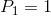Please show all work: Let If x is odd then If x is even then Prove that is true and then solve it. We were unable to transcribe this imageWe were unable to transcribe this imageWe were unable to transcribe this imageWe were unable to transcribe this image

• ### When px^3 – 2px^2 + 3x – p is divided by (x – 1), the remainder is -5

When px^3 – 2px^2 + 3x – p is divided by (x – 1), the remainder is -5. Find p.

• ### When px^3 – 2px^2 + 3x – p is divided by (x – 1), the remainder is -5

When px^3 – 2px^2 + 3x – p is divided by (x – 1), the remainder is -5. Find p. (2 marks)

• ### Show all work please. 1. U(x, y) x,ax,1-a) a. Solve for the marshallian demands for x,...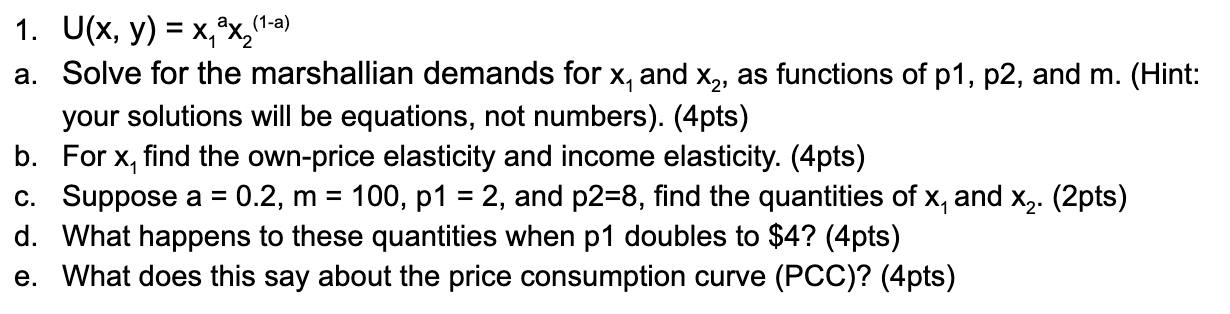Show all work please. 1. U(x, y) x,ax,1-a) a. Solve for the marshallian demands for x, and x2, as functions of p1, p2, and m. (Hint: your solutions will be equations, not numbers). (4pts) b. For x, find the own-price elasticity and income elasticity. (4pts) c. Suppose a = d. What happens to these quantities when p1 doubles to \$4? (4pts) e. What does this say about the price consumption curve (PCC)? (4pts) 100, p1 2, and p2=8, find the...

• ### The demand function for good X is as follows: X= 25 + 5Py+ 5B-2PX A. What...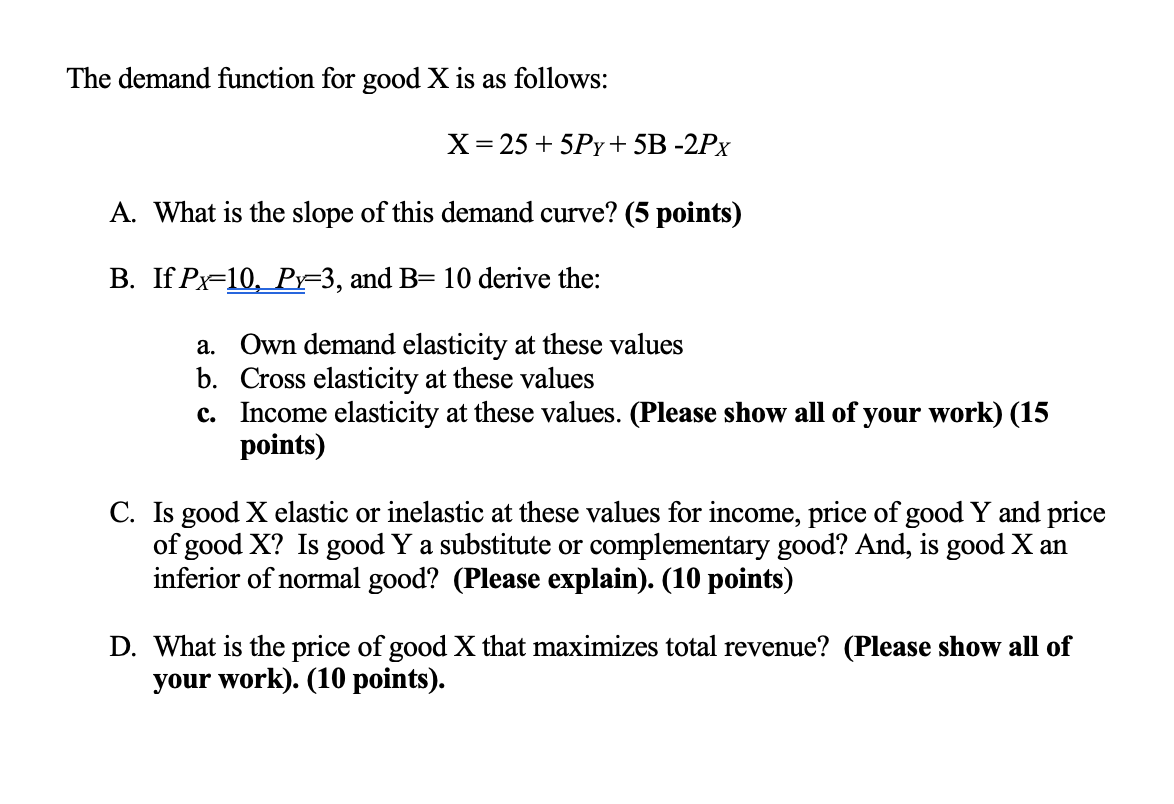The demand function for good X is as follows: X= 25 + 5Py+ 5B-2PX A. What is the slope of this demand curve? (5 points) B. If Px=10, Py=3, and B= 10 derive the: a. Own demand elasticity at these values b. Cross elasticity at these values C. Income elasticity at these values. (Please show all of your work) (15 points) C. Is good X elastic or inelastic at these values for income, price of good Y and price of...

• ### PLEASE SHOW WORK Question 3 Use mathematical induction to prove 3+7+11+ ... +(4n – 1) =...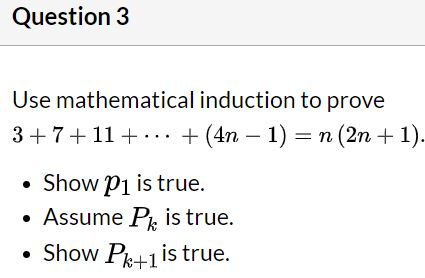PLEASE SHOW WORK Question 3 Use mathematical induction to prove 3+7+11+ ... +(4n – 1) = n (2n + 1). • Show P1 is true. • Assume Pk is true. • Show Pk+1 is true.

• ### Please show all work Let f(x) = x2 + 3x + 5. a) Find all derivatives...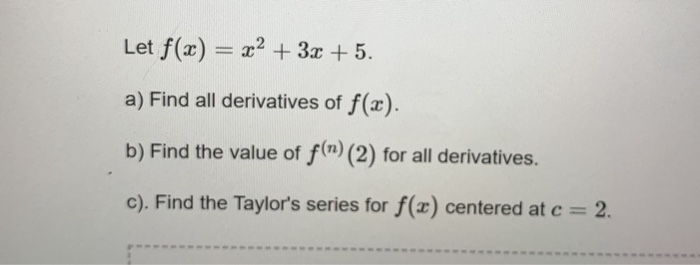Please show all work Let f(x) = x2 + 3x + 5. a) Find all derivatives of f(x). b) Find the value of f(n) (2) for all derivatives. c). Find the Taylor's series for f(x) centered at c = 2.

• ### Discrete math show all work please Use mathematical induction to prove that the statements are true...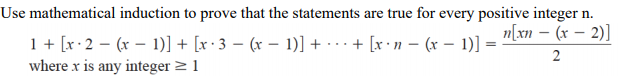Discrete math show all work please Use mathematical induction to prove that the statements are true for every positive integer n. n[xn - (x - 2)] 1 + [x2 - (x - 1)] + [x:3 - (x - 1)] + ... + x n - (x - 1)] = 2 where x is any integer = 1